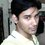# SoLn.

Our Problem can be classified as an indirect problem of kinematics. Thus from (1.3) & (1.2) we have to move towards (1.1) .It is of utmost importance in any kind of problem to know your direction (even if you don't know the path.)

Using (1.3)

$\cfrac { dv }{ dt } =a \\ dv=adt \\ \int _{ u }^{ v }{ dv } =\int _{ 0 }^{ t }{ adt }$

As the time changes from 0 to t the velocity changes from u to v.So on the left hand side the summation is made over v from u to v whereas on the right hand side the summation is made on time from 0 to t. Evaluating the integrals we get

${ [v] }_{ u }^{ v }=a{ [t] }_{ 0 }^{ t } \\ v-u=at \\ v=u+at$

Using (1.2) the last equation may be written as

$\cfrac { dx }{ dt } =v=u+at \\ dx=(u+at)dt \\ \int _{ 0 }^{ x }{ dx } =\int _{ 0 }^{ t }{ (u+at)dt }$

At t=0 the particle is at x=0. As time changes from 0 to t the position changes from 0 to x.So on the left hand side the summation is made on position from 0 to x whereas on the right hand side the summation is made on time from 0 to t.Evaluating the integrals we get,

${ [x] }_{ 0 }^{ x }=\int _{ 0 }^{ t }{ udt } \int _{ 0 }^{ t }{ atdt } \\ x=u{ [t] }_{ 0 }^{ t }+a{ { [t }^{ 2 }/2] }_{ 0 }^{ t } \\ x=ut+(a{ t }^{ 2 })/2$

Using the above two derived expressions,

${ v }^{ 2 }={ (u+at) }^{ 2 } \\ ={ u }^{ 2 }+2uat+{ a }^{ 2 }{ t }^{ 2 } \\ ={ u }^{ 2 }+2a[ut+\cfrac { 1 }{ 2 } a{ t }^{ 2 }] \\ ={ u }^{ 2 }+2ax$

The equations

$v=u+at$

$x=ut+\frac { 1 }{ 2 } a{ t }^{ 2 }$

${ v }^{ 2 }={ u }^{ 2 }+2ax$

are used very frequently while solving problems in kinematics involving constant acceleration as one of the physical quantities.If,however,the acceleration isn't constant,these three equations are not useful.We, then, need other tools & procedures.Note by Soumo Mukherjee
6 years, 7 months ago

This discussion board is a place to discuss our Daily Challenges and the math and science related to those challenges. Explanations are more than just a solution — they should explain the steps and thinking strategies that you used to obtain the solution. Comments should further the discussion of math and science.

When posting on Brilliant:

• Use the emojis to react to an explanation, whether you're congratulating a job well done , or just really confused .
• Ask specific questions about the challenge or the steps in somebody's explanation. Well-posed questions can add a lot to the discussion, but posting "I don't understand!" doesn't help anyone.
• Try to contribute something new to the discussion, whether it is an extension, generalization or other idea related to the challenge.
• Stay on topic — we're all here to learn more about math and science, not to hear about your favorite get-rich-quick scheme or current world events.

MarkdownAppears as
*italics* or _italics_ italics
**bold** or __bold__ bold
- bulleted- list
• bulleted
• list
1. numbered2. list
1. numbered
2. list
Note: you must add a full line of space before and after lists for them to show up correctly
paragraph 1paragraph 2

paragraph 1

paragraph 2

[example link](https://brilliant.org)example link
> This is a quote
This is a quote
    # I indented these lines
# 4 spaces, and now they show
# up as a code block.

print "hello world"
# I indented these lines
# 4 spaces, and now they show
# up as a code block.

print "hello world"
MathAppears as
Remember to wrap math in $$ ... $$ or $ ... $ to ensure proper formatting.
2 \times 3 $2 \times 3$
2^{34} $2^{34}$
a_{i-1} $a_{i-1}$
\frac{2}{3} $\frac{2}{3}$
\sqrt{2} $\sqrt{2}$
\sum_{i=1}^3 $\sum_{i=1}^3$
\sin \theta $\sin \theta$
\boxed{123} $\boxed{123}$

## Comments

There are no comments in this discussion.

×

Problem Loading...

Note Loading...

Set Loading...